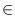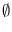Next: Sets of Parallel Segments Up: Advanced Data Types for Previous: Point Location in Triangulations   Contents   Index

# Sets of Intervals ( interval_set )

Definition

An instance S of the parameterized data type interval_set<I> is a collection of items ( isitem). Every item in S contains a closed interval of the double numbers as key and an information from data type I, called the information type of S. The number of items in S is called the size of S. An interval set of size zero is said to be empty. We use < x, y, i > to denote the item with interval [x, y] and information i; x (y) is called the left (right) boundary of the item. For each interval [x, y] there is at most one item < x, y, i >S.

#include < LEDA/geo/interval_set.h >

Creation

 interval_set S creates an instance S of type interval_set and initializes S to the empty set.

Operations

 double S.left(is_item it) returns the left boundary of item it. Precondition it is an item in S. double S.right(is_item it) returns the right boundary of item it. Precondition it is an item in S. const I& S.inf(is_item it) returns the information of item it. Precondition it is an item in S. is_item S.insert(double x, double y, const I& i) associates the information i with interval [x, y]. If there is an item < x, y, j > in S then j is replaced by i, else a new item < x, y, i > is added to S. In both cases the item is returned. is_item S.lookup(double x, double y) returns the item with interval [x, y] (nil if no such item exists in S). list const S.intersection(double a, double b) returns all items < x, y, i >S with [x, y][a, b]! =. void S.del(double x, double y) deletes the item with interval [x, y] from S. void S.del_item(is_item it) removes item it from S. Precondition it is an item in S. void S.change_inf(is_item it, const I& i) makes i the information of item it. Precondition it is an item in S. void S.clear() makes S the empty interval_set. bool S.empty() returns true iff S is empty. int S.size() returns the size of S.

Implementation

Interval sets are implemented by two-dimensional range trees [90,57]. Operations insert, lookup, del_item and del take time O(log2n), intersection takes time O(k + log2n), where k is the size of the returned list. Operations left, right, inf, empty, and size take time O(1), and clear O(n log n). Here n is always the current size of the interval set. The space requirement is O(n log n).Next: Sets of Parallel Segments Up: Advanced Data Types for Previous: Point Location in Triangulations   Contents   Index